# Vasquez and Beggs Oil Compressibility correlation

Jump to: navigation, search

## Vasquez and Beggs Oil Compressibility correlation

Vasquez and Beggs is an empirical correlation for the oil compressibility published in 1980.

## Math & Physics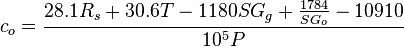$c_o = \frac{28.1R_s+30.6T-1180SG_g+\frac{1784}{SG_o}-10910}{10^5P}$

## Example. Calculation of the oil compressibility

Example source 

### Input data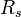$R_s$ = 53.24 sm3/sm3 at P = 10 MPa$T$ = 90C or 363K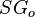$SG_o$ = 0.85 or 35 API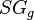$SG_g$ = 0.75

Calculate oil compressibility at p = 10 MPa?

### Solution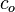$c_o$ = 0.002907 MPa-1 or 2.95E-4 atm

The solution is available in the online PVT calculator software model at www.pengtools.com

## Nomenclature$c_o$ = oil compressibility, MPa-1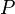$P$ = pressure, MPa$R_s$ = solution gas-oil ratio, sm3/sm3$SG_g$ = gas specific gravity, dimensionless$SG_o$ = oil specific gravity, dimensionless$T$ = temperature, °K